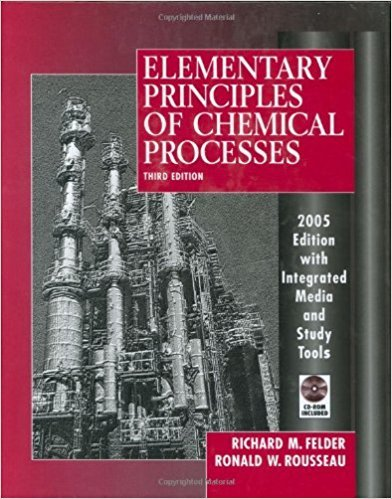×
Get Full Access to Elementary Principles Of Chemical Processes - 3 Edition - Chapter 10 - Problem 10.2
Get Full Access to Elementary Principles Of Chemical Processes - 3 Edition - Chapter 10 - Problem 10.2

×

# Benzene (B) and chlorobenzene (C) are being separated in aISBN: 9780471687573 143

## Solution for problem 10.2 Chapter 10

Elementary Principles of Chemical Processes | 3rd Edition

• Textbook Solutions
• 2901 Step-by-step solutions solved by professors and subject experts
• Get 24/7 help from StudySoup virtual teaching assistantsElementary Principles of Chemical Processes | 3rd Edition

4 5 1 248 Reviews
20
0
Problem 10.2

Benzene (B) and chlorobenzene (C) are being separated in a distillation column. Vapor and liquid streams, each containing both species, are fed to one of the trays of the column, and liquid and vapor streams are taken off the tray. The tray functions as an ideal stage (see 6.63): the effluent streams are in equilibrium at temperature T and pressure P, with compositions related by Raoult's law, Equation 6.4-1. nl(mal vapor) n3(mol vapor) Xl (mal S/mol) IDEAL x3(mol S/mol) } Eq";lib,;"m .t n2(mol liquid) STAGE n4(mol liquid) T.P x2(mol S/mol) x4(mol S/mol) Calculate the number of degrees of freedom. Then specify sets of design variables for which the solution for the remaining state variables would be (a) straightforward, (b) iterative, and (c) impossible.

Step-by-Step Solution:
Step 1 of 3

Chemistry Notes Electron Configurations  Rule 1: Electrons fill lowest sublevels first (Aufbau Principle) & fill in the order of the PT  Rule 2: Each orbital can hold a maximum of two electrons which must be in opposite spin (Pauli Exclusion Principle) ↿ ⇂  Electrons don’t pair up within a sublevel until there are no empty orbitals (Hund’s Rule) ↿ ⇂ ↿ Radioactivity  Alpha Particle= Helium nucleus; 2 protons, 2 neutrons, mass is 4, stopped by paper  Gamma Ray= Beam of light; 0 charge, 0 mass, stopped by lead  Beta Particle= Electron; mass is 1/2000, charge is -1, stopped by aluminum Formation of Ions  Ion- electrically charged atom or group of atoms  Cation- positively charge

Step 2 of 3

Step 3 of 3

##### ISBN: 9780471687573

This textbook survival guide was created for the textbook: Elementary Principles of Chemical Processes, edition: 3. This full solution covers the following key subjects: mol, liquid, vapor, Streams, column. This expansive textbook survival guide covers 13 chapters, and 710 solutions. Since the solution to 10.2 from 10 chapter was answered, more than 273 students have viewed the full step-by-step answer. The answer to “Benzene (B) and chlorobenzene (C) are being separated in a distillation column. Vapor and liquid streams, each containing both species, are fed to one of the trays of the column, and liquid and vapor streams are taken off the tray. The tray functions as an ideal stage (see 6.63): the effluent streams are in equilibrium at temperature T and pressure P, with compositions related by Raoult's law, Equation 6.4-1. nl(mal vapor) n3(mol vapor) Xl (mal S/mol) IDEAL x3(mol S/mol) } Eq";lib,;"m .t n2(mol liquid) STAGE n4(mol liquid) T.P x2(mol S/mol) x4(mol S/mol) Calculate the number of degrees of freedom. Then specify sets of design variables for which the solution for the remaining state variables would be (a) straightforward, (b) iterative, and (c) impossible.” is broken down into a number of easy to follow steps, and 123 words. Elementary Principles of Chemical Processes was written by and is associated to the ISBN: 9780471687573. The full step-by-step solution to problem: 10.2 from chapter: 10 was answered by , our top Chemistry solution expert on 11/15/17, 02:42PM.

Unlock Textbook Solution